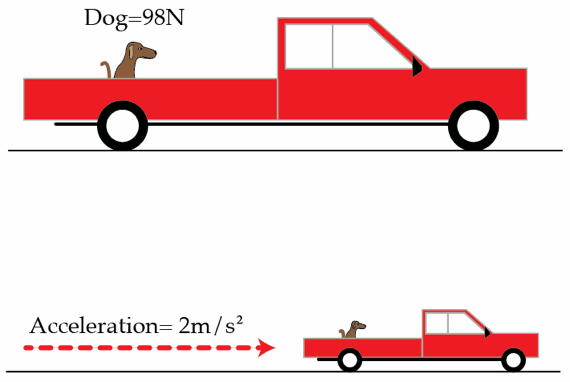# Keep the dog safe

A dog with weight of $98~\mbox{N}$ sits in the back of a pickup truck, which is initially at rest. After some time the truck accelerates to the right with an acceleration of $2~\mbox{m/s}^2$. The dog does not begin to slide with respect to the truck bed as the truck accelerates. What is the force of friction on the dog in Newtons?Details and assumptions

• The acceleration of gravity is $-9.8~\mbox{m/s}^2$.
• The coefficient of static friction between the dog and the truck bed is $0.8$.
×